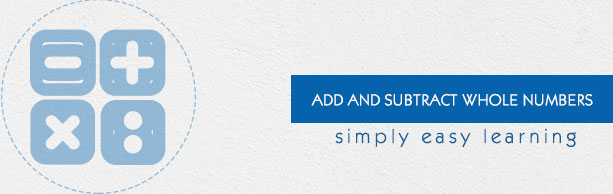# Add and Subtract Whole Numbers

This tutorial provides comprehensive coverage of addition and subtraction of whole numbers based on Common Core (CCSS) and State Standards and its prerequisites. Students can navigate learning paths based on their level of readiness. Institutional users may customize the scope and sequence to meet curricular needs. This simple tutorial uses appropriate examples to help you understand addition and subtraction of whole numbers in a general and quick way.

# Audience

This tutorial has been prepared for beginners to help them understand the basics of Addition and subtraction of whole numbers. After completing this tutorial, you will find yourself at a moderate level of expertise in addition and subtraction of whole numbers, from where you can advance further.

# Prerequisites

Before proceeding with this tutorial, you need a basic knowledge of elementary math concepts such Number Sense, Addition, Subtraction, etc.